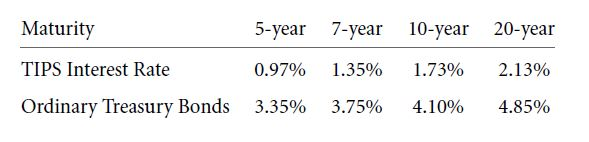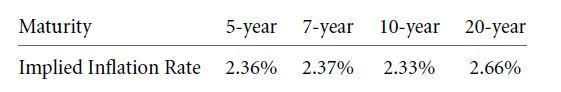Let us take an example to understand this. Say, in case of a two-year investment, the rate of interest for the first year is 5% and it is 10% for the second year. The forward rate will be equivalent to 15.24%. Now, two explanations can be given:

1. The 1-year rate of interest will be higher in the next year. The 1-year rate of interest in the next year might even be higher than 15.24%.
2. Investors earn high rates of return by holding long-term bonds than by holding short-term bonds. If the yield curve does not have an upward slope, then the value of interest the next year will be lower.

Let us know study whether higher present rates of interest will ensure higher rates of interest in the future.

5.4 A DOES IT PREDICT HIGHER FUTURE INFLATION?

Generally, when inflates is high, the nominal interest rates are bound to go higher and vice-versa.

The upward slope of the yield-curve can be explained in terms of inflation. Say, the rates of interest are high in the upcoming future but there is also a probability of inflation.

Since 1997, Treasury has been selling bonds that are inflation-indexed. They promise to pay the interest rate plus the promised CPI inflation rate. These are known as Treasury Inflation Protected Securities (TIPS). They are sometimes also known as CPI bonds. These are not affected by inflation. Even if the nominal yield curve is upward sloping due to high rates of inflation, the yield curve of TIPS does not have an upward slope.

The scenario of the Treasury in December 2004 is depicted below:Here the TIPS yield curve is upward sloping. So, we can say that higher future expected inflation rates are not the only reason why yield curves are upward sloping.

The implied interest rate, when calculated, comes out to be:In 2004, it could have been said that a 3-basis point would be the reason for inflation from 2014 to 2024. Higher inflation rates might have a role to play in future.

REVIEW QUESTION

1. A 30-year inflation adjusted bond offers a real yield of 3.375% per year. Given the current inflation rate of 1.6% per year, a 30-year bond offering a nominal yield of 5.6% per year, but it is not inflation adjusted. Which one will you buy and buy?

5.4 B   DOES IT PREDICT HIGHER FUTURE INTEREST RATE?

As short term rates will be higher in future, therefore, yield-curves are upward sloping. It is predicted that in future, capital will be scarce but investment opportunities will be better and this will lead to a higher interest rate.

Unfortunately, though, future inflation rates can be estimated but there is no way to estimate future interest rate.

5.4 C DOES YIELD CURVE COMPENSATE THE RISK?

Long term bonds yielded more than short term bonds. But, long term bonds are riskier than short-term bonds and therefore, they need to offer more return on average so that investors choose the long term bonds. This is the only way by which the risk factor can be compensated.

Links of Next Financial Accounting Topics:-### Customer Reviews

My Homework Help
Rated 5.0 out of 5 based on 510 customer reviews at